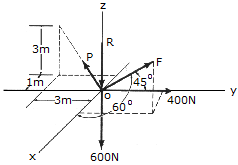# Engineering Mechanics - Equilibrium of a Particle - Discussion

### Discussion :: Equilibrium of a Particle - General Questions (Q.No.3)

3.Determine the magnitudes ofthe forces P, R, and F required for equillibrium of point O.

 [A]. R = 238 N, F = 181.0 N, P = 395 N [B]. R = 1340 N, F = 740 N, P = 538 N [C]. R = 419 N, F = 181.0 N, P = 395 N [D]. R = 409 N, F = 504 N, P = 1099 N

Explanation:

No answer description available for this question.

 P.Velmurugan said: (Sep 30, 2011) A body of weight 100N is suspended by two strings of 4 M and 3 M lengths attached at the same horizontal level 5 M apart. Find the tension of strings.

 P.Velmurugan said: (Sep 30, 2011) A) A t section has flange 200x20mm and web 15x240mm. Overall depth is260mm. Find the position of the centroid. B) A solid cone having base diameter 8 cm and height 6cm is kept co-axially on a solid cylinder having 8cm diameter and 10cm height. find the C.G of the combination.

 Gaurav Kumar said: (Jan 23, 2013) It is a very simple question involves basic knowledge. Use the concept of vector. In this question use the formulas for force making angle with all three axis i.e. the cosine one. Find vector F, vector OP and write other forces in vector form. Since particle is in equilibrium add all forces and equate to 0.

 Manuel Ziwu said: (Oct 30, 2013) Thank you. @Gaurav Kumar. But how can I find the one for the z-axis. That is for vector F, I could only find the angles for the x and y- axes. Thank you in advance.

 Kaliraj said: (Aug 10, 2020) How to identify, which plane has which forces? I am confusing to take forces in the right plane. Please anyone explain me.